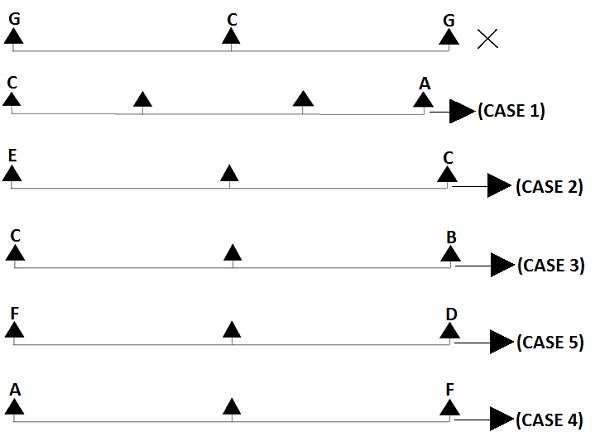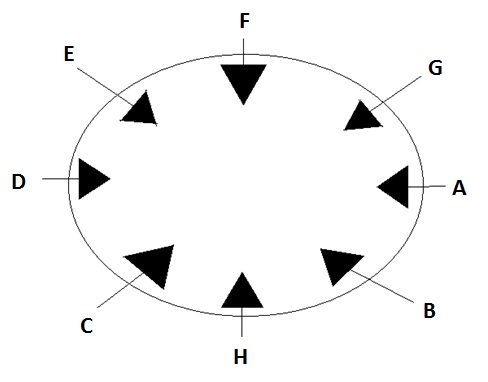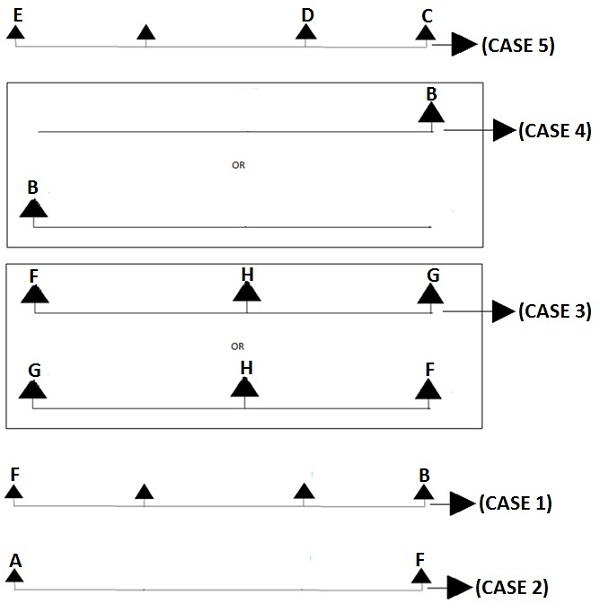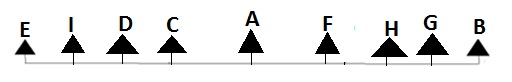# Seating Arrangement - Solved Examples

Question: Study the given information carefully and answer the following question. Eight men A, B, C, D, E, F, G and H are sitting around a circular table facing the centre for having their lunch.

(1) G is not an immediate neighbor of C.

(2) A is third to the right of C.

(3) E is second to the left of C.

(4) C is second to the left of B.

(5) F is second to the left of D.

(6) A is second to the left of F.

Q 1 − Who is sitting to the immediate right of C?

Options :

A - G

B - F

C - D

D - H

ExplanationBy applying (CASE 1), (CASE 2), (CASE 3), (CASE 4) and (CASE 5), we getBy observing the diagram, we can clearly say that H is sitting to the immediate right of C.

Question: Study the given information carefully and answer the following question. Eight men A, B, C, D, E, F, G and H are sitting around a circular table facing the centre for having their lunch.

(1) G is not an immediate neighbor of C.

(2) A is third to the right of C.

(3) E is second to the left of C.

(4) C is second to the left of B.

(5) F is second to the left of D.

(6) A is second to the left of F.

Q 2 − Who is sitting to the immediate left of H?

Options :

A - E

B - G

C - A

D - C

Explanation - According to the diagramBy observing the diagram, we can conclude that C is sitting to the immediate left of H.

Question: Study the given information carefully and answer the following question. Eight men A, B, C, D, E, F, G and H are sitting around a circular table facing the centre for having their lunch.

(1) G is not an immediate neighbor of C.

(2) A is third to the right of C.

(3) E is second to the left of C.

(4) C is second to the left of B.

(5) F is second to the left of D.

(6) A is second to the left of F.

Q 3 − What is the position of D with respect to H?

Options :

A - Third to the left.

B - Second to the left.

C - Immediate next.

D - Fifth to the left.

Explanation - According to the diagramTo the immediate left of H, C is sitting and in the second left position D is sitting. So the position of D is second to the left of H.

Question: Study the given information carefully and answer the following question. Eight men A, B, C, D, E, F, G and H are sitting around a circular table facing the centre for having their lunch.

(1) G is not an immediate neighbor of C.

(2) A is third to the right of C.

(3) E is second to the left of C.

(4) C is second to the left of B.

(5) F is second to the left of D.

(6) A is second to the left of F.

Q 4 − Which of the following statements is true?

Options :

A - H is sitting between A and G.

B - H is to the immediate left of F.

C - D is sitting fifth to the left of H.

D - H is sitting between C and B.

Explanation - According to the diagramBy observing the diagram, we can conclude that (A), (B) and (C) do not satisfy the given sitting arrangement in the diagram. But option (D) does.

Question: Study the given information carefully and answer the following question. Eight men A, B, C, D, E, F, G and H are sitting around a circular table facing the centre for having their lunch.

(1) G is not an immediate neighbor of C.

(2) A is third to the right of C.

(3) E is second to the left of C.

(4) C is second to the left of B.

(5) F is second to the left of D.

(6) A is second to the left of F.

Q 5 − Which of the following is the first person sitting between the second and third person?

Options :

A - ABG

B - DCH

C - CHB

D - FED

Explanation - According to the diagramBy observing the sitting arrangement, we can say that option (A) satisfies the condition. A is sitting between the second person B and third person G which is our required answer.

Question: Study the given information carefully and answer the following question. Eight men A, B, C, D, E, F, G and H are sitting around a circular table facing the centre for having their lunch.

(1) G is not an immediate neighbor of C.

(2) A is third to the right of C.

(3) E is second to the left of C.

(4) C is second to the left of B.

(5) F is second to the left of D.

(6) A is second to the left of F.

Q 6 − Among the eight men which two men are not neighbor?

Options :

A - CH

B - BA

C - DA

D - EF

Explanation - According to the diagramBy observing the diagram, we can conclude that D and A are not neighbor. Rather D is sitting fourth to the left of A if counted in clockwise direction.

Question: Study the given information carefully and answer the following question.

Nine girls A, B, C, D, E, F, G, H and I are seated in a row for watching movie. D is sitting second to the right of E and C is sitting third to the right of E. B is sitting at one end of the row. H is sitting between F and G. B is sitting third to the right of F. F is sitting to the immediate right of A?

Q 7 − What is the position of I?

Options :

A - To the immediate right of B.

B - To the immediate left of G.

C - Sitting between A and H.

D - Sitting between E and D.

ExplanationBy applying (CASE 1), (CASE 2), (CASE 3), (CASE 4) and (CASE 5), we get the following sitting arrangements.By observing the diagram, one can clearly say that I is sitting between E and D.

Question: Study the given information carefully and answer the following question.

Nine girls A, B, C, D, E, F, G, H and I are seated in a row for watching movie. D is sitting second to the right of E and C is sitting third to the right of E. B is sitting at one end of the row. H is sitting between F and G. B is sitting third to the right of F. F is sitting to the immediate right of A?

Q 8 − Which of the following is the first girl sitting between the second and the third girl?

Options :

A - GHB

B - CAF

C - EID

D - DAC

Explanation - According to the diagramHere in the option (A), the first girl G is sitting between the second girl H and the third girl B. So our required answer is GHB.

Question: Study the given information carefully and answer the following question.

Nine girls A, B, C, D, E, F, G, H and I are seated in a row for watching movie. D is sitting second to the right of E and C is sitting third to the right of E. B is sitting at one end of the row. H is sitting between F and G. B is sitting third to the right of F. F is sitting to the immediate right of A?

Q 9 − Which of the following pairs represents the immediate neighbors of A?

Options :

A - EI

B - CF

C - GB

D - DI

Explanation - According to the diagramA is sitting between the two girls C and F. Therefore our required answer goes to the pair CF.

Question: Study the given information carefully and answer the following question.

Nine girls A, B, C, D, E, F, G, H and I are seated in a row for watching movie. D is sitting second to the right of E and C is sitting third to the right of E. B is sitting at one end of the row. H is sitting between F and G. B is sitting third to the right of F. F is sitting to the immediate right of A?

Q 10 − Who is sitting to the right of E?

Options :

A - A

B - B

C - I

D - G

Explanation - According to the diagramIt is clear that the girl I is sitting to the immediate right of E. Therefore our required answer is option C.

Question: Study the given information carefully and answer the following question.

Nine girls A, B, C, D, E, F, G, H and I are seated in a row for watching movie. D is sitting second to the right of E and C is sitting third to the right of E. B is sitting at one end of the row. H is sitting between F and G. B is sitting third to the right of F. F is sitting to the immediate right of A?

Q 11 − Which girl is sitting exactly in the middle of the all girls?

Options :

A - A

B - B

C - E

D - None of these

Explanation - According to the diagramThere are total nine girls. From the nine girls, four girls which are E, I, D and C are sitting to the left of A and F,H,G and B are the other four girls sitting to the right of A. Therefore A sits on the middle of all the girls.

Question: Study the given information carefully and answer the following question.

Nine girls A, B, C, D, E, F, G, H and I are seated in a row for watching movie. D is sitting second to the right of E and C is sitting third to the right of E. B is sitting at one end of the row. H is sitting between F and G. B is sitting third to the right of F. F is sitting to the immediate right of A?

Q 12 − How many girls are seated between E and H?

Options :

A - Three

B - Four

C - Five

D - Six

Explanation - According to the diagramFive girls I, D, C, A, and F are sitting between E and H.

reasoning_seating_arrangement.htm